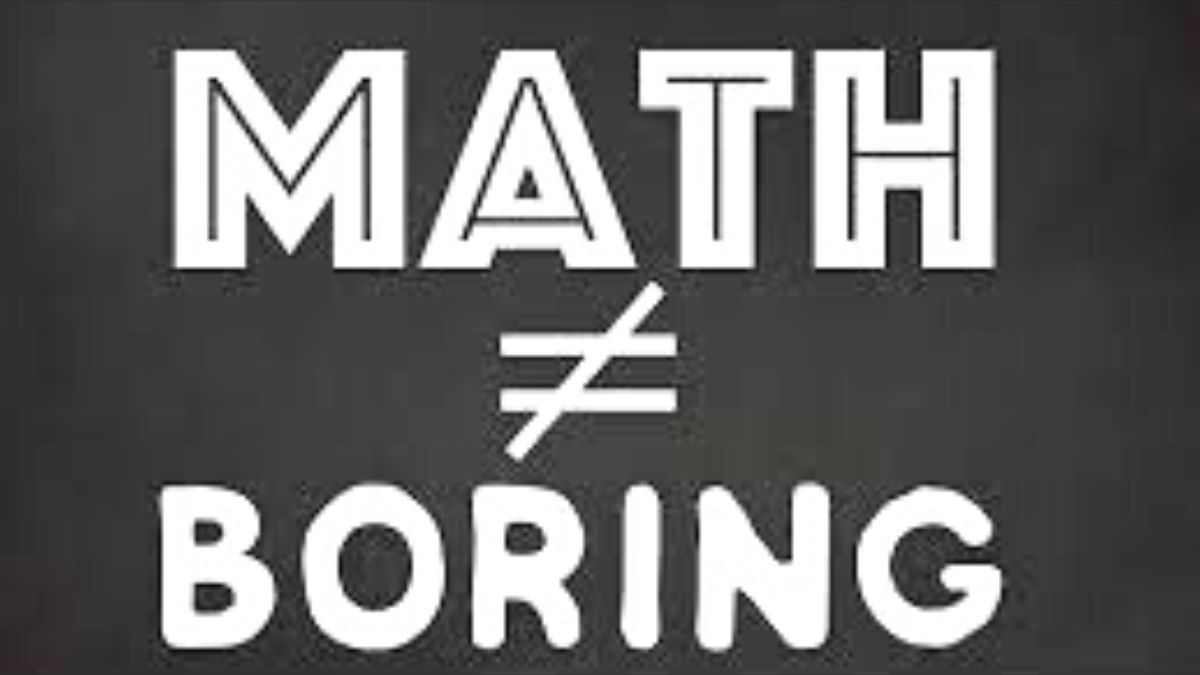# Math is not always boring! These math riddles will make you love the subject!

Math is always considered boring and tough. Here we try to change your harsh perception of math with these exciting math riddles.Math has always been the most hated subject among students. Many students secretly like the subject, but they are scared by its theorems. And oh, the pressure to score well is unmatched.

Here we bring to you some exciting math riddles to try!

Math Riddle 1:

Here comes a tough task. You need to make use of just a single letter in order to reach 720 from 98. Can you do that?

Math Riddle 2:

You are at a fruit mart. However, you forgot to carry your calculator for the calculation. Now, you need to prove your mental math skills here. A pear is 40 cents, an apricot is 60 cents and a watermelon is 80 cents. How much is an apple?

Math Riddle 3:

How many 9’s do you think are there between 1 and 100?

Math Riddle 1:

Here comes a tough task. You need to make use of just a single letter in order to reach 720 from 98. Can you do that?

You need to simply add an “x: between 90 and 8. 90 multiplied by 8 is 720.

Math Riddle 2:

You are at a fruit mart. However, you forgot to carry your calculator for the calculation. Now, you need to prove your mental math skills here. A pear is 40 cents, an apricot is 60 cents and a watermelon is 80 cents. How much is an apple?

40 cents. How? Well, the price of the fruits is actually calculated by multiplying the number of vowels by 20c, in this problem.

Math Riddle 3:

How many 9’s do you think are there between 1 and 100?